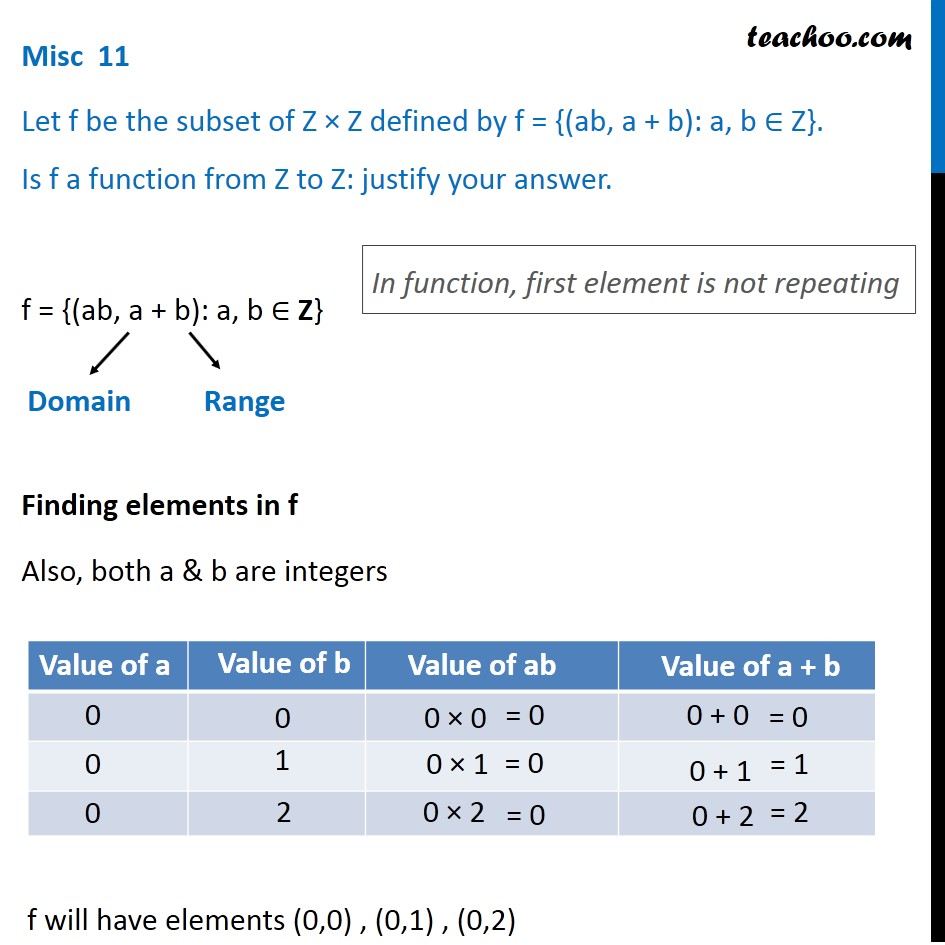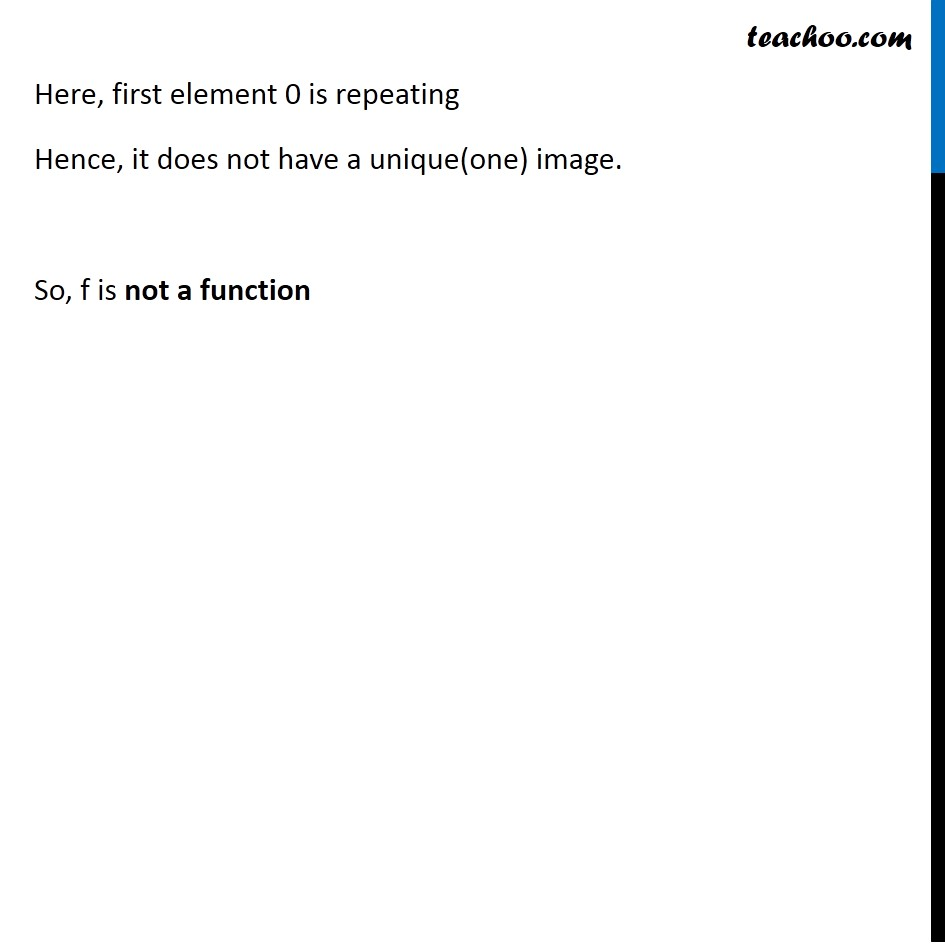1. Chapter 2 Class 11 Relations and Functions (Term 1)
2. Serial order wise
3. Miscellaneous

Transcript

Misc 11 Let f be the subset of Z × Z defined by f = {(ab, a + b): a, b ∈ Z}. Is f a function from Z to Z: justify your answer. f = {(ab, a + b): a, b ∈ Z} Finding elements in f Also, both a & b are integers In function, first element is not repeating f will have elements (0,0) , (0,1) , (0,2) Here, first element 0 is repeating Hence, it does not have a unique(one) image. So, f is not a function

Miscellaneous

Chapter 2 Class 11 Relations and Functions (Term 1)
Serial order wise